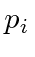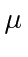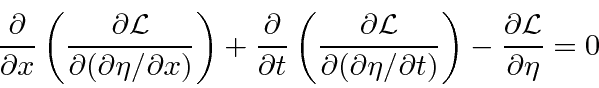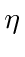## Simple Mechanical Systems and Fields

This section is a review of mechanical systems largely from the point of view of Lagrangian dynamics. In particular, we review the equations of a string as an example of a field theory in one dimension.

We start with the Lagrangian of a discrete system like a single particle.Lagrange's equations arewhere theare the coordinates of the particle. This equation is derivable from the principle of least action.Similarly, we can define the Hamiltonianwhereare the momenta conjugate to the coordinates.For a continuous system, like a string, the Lagrangian is an integral of a Lagrangian density function.For example, for a string,whereis Young's modulus for the material of the string andis the mass density. The Euler-Lagrange Equation for a continuous system is also derivable from the principle of least action states above. For the string, this would be.Recall that the Lagrangian is a function ofand its space and time derivatives.

The Hamiltonian density can be computed from the Lagrangian density and is a function of the coordinateand its conjugate momentum.In this example of a string,is a simple scalar field. The string has a displacement at each point along it which varies as a function of time.

If we apply the Euler-Lagrange equation, we get a differential equation that the string's displacement will satisfy.This is the wave equation for the string. There are easier ways to get to this wave equation, but, as we move away from simple mechanical systems, a formal way of proceeding will be very helpful.

Jim Branson 2013-04-22